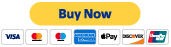## PRICING

Percentage Pricing System

Pricing for Estimates

\$0 - \$99,999 = 1% of the total

\$100,000 - \$199,000 = .85% of the total

\$200,000 - \$299,000 = .75% of the total

\$300,000 + = .50% of the total

To start a project you must pay the \$89.00 minimum. No project can be started without the minimum payment.

This is the minimum charge for
any estimate and covers an estimate up to \$8,999.99.

If your estimate exceeds \$8,999.00 you will receive an invoice for the difference.
Forexample if the estimate totals \$15,000 the cost of the estimate is \$150.00. You will receive and invoice for \$150 - \$89 initial payment = \$61.00. Upon receipt of payment you will receive the finalized invoice.

If your estimate is under \$8,999.99 you will receive the finalizes estimate via email.

## Pay Deposit to Start an Estimate Now -->